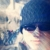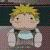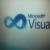## 数据量大时（约千个左右）会很卡。

@Kener-林峰 你好，想跟你请教个问题：新手上路,使用折线图，X轴从数据库取值，大约有2000个点，对应Y轴也有这么多数据量，会导致图载入很慢，IE显示脚 本停止。直接在X轴配置上添加的。

2013-12-21补充内容：

```while(rs1.next()){
BigDecimal temp=rs1.getBigDecimal(tempstr[j]);
BigDecimal df=temp.setScale(1, BigDecimal.ROUND_HALF_UP);
datetemp = rs1.getTime(1);
String outtemp=df.toString();
if (timestring.equals(" ")) {
timestring="['"+datetemp+"'";
}else{
timestring=timestring+",'"+datetemp+"'";
}

if (datestring.equals(" ")) {
datestring="['"+outtemp+"'";
}else{
datestring=datestring+",'"+outtemp+"'";
}
}
}
timestring=timestring+"]";
datestring=datestring+"]";```

JS部份

```xAxis : [
{
type : 'category',
boundaryGap : false,
data :<%=timestring%>
}
],
yAxis : [
{
type : 'value',
splitArea : {show : true}
}
],
series : [
{
name:'33',
type:'line',
stack: '总量',
data:<%=datestring%>

}

]```

0option.animation : false//动画

option.calculable:false //拖拽重计算

0
d

000

0

0

#### 引用来自“Kener-林峰”的答案

```],
series : [
{
name:'33',
type:'line',
stack: '总量',
data:<%=datestring%>

}

],

animation:false
};```